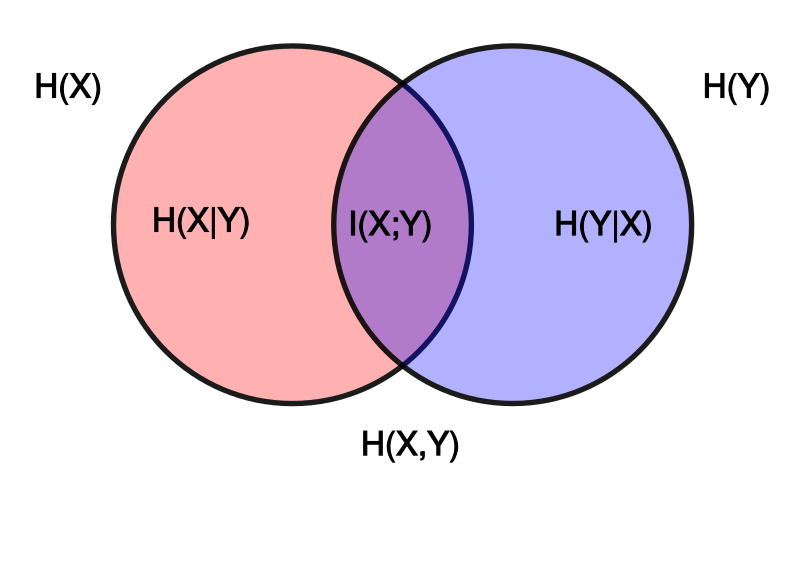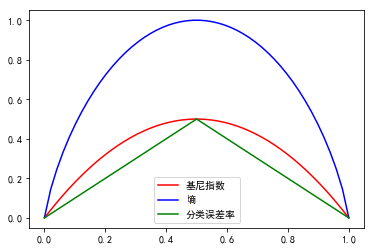2019/07/14

# 决策树

## 特征选择

### 信息增益

1. 定义

假设训练样本集合为$D$，$\left| D \right|$表示集合中的样本个数。其中有$K$个类$C_k,k=1,2,\cdots,K, \left| C_k \right|$表示类别$C_k$中的样本个数，且有$\sum_{k=1}^K{\left| C_k \right|}=\left| D \right|$。设某个特征$A$有$n$个不同的取值$\left\{ a_1,a_2,\cdots,a_n \right\}$,根据特征$A$的不同取值将$D$划分为$n$个子集$D_1、D_2、\cdots D_n,\left| D_i \right|$表示$D_i$的样本个数，且有$\sum_{i=1}^n{\left| D_i \right|}=\left| D \right|$。记子集$D_i$中属于类$C_k$的样本的集合为$D_ik$,$\left| D_ik \right|$表示$D_ik$的样本个数。

则数据集$D$的经验熵为

特征$A$对数据集$D$的经验条件熵为

特征$A$对训练数据集$D$的信息增益为

【补充: 熵-联合熵-条件熵 关系及推导】

设$X,Y$是两个变量集合，$x,y$分别是$X,Y$中的具体元素，变量集合的熵-联合熵-条件熵(衡量信息的不确定性，值越大，不确定性越大)的关系如图所示左边的椭圆代表$H(X)$,右边的椭圆代表$H(Y)$,中间重合的部分代表互信息或者信息增益$I(X,Y)$, 左边的椭圆去掉重合部分代表$H(X|Y)$,右边的椭圆去掉重合部分代表$H(Y|X)$。两个椭圆的并集代表$H(X,Y)$。

具体地

$=\sum_{x\in X}{p\left( x \right) \log p\left( x \right)}-\sum_{x\in X}{\sum_{y\in Y}{p\left( x,y \right) \log p\left( x,y \right)}}$ $=\sum_{x\in X}{p\left( x \right) \log p\left( x \right)}-\sum_{x\in X}{\sum_{y\in Y}{p\left( x,y \right) \left( \log p\left( x \right) +\log p\left( y\left\| x \right. \right) \right)}}$ $=\sum_{x\in X}{p\left( x \right) \log p\left( x \right)}-\sum_{x\in X}{\sum_{y\in Y}{p\left( x,y \right) \log p\left( x \right)}}-\sum_{x\in X}{\sum_{y\in Y}{p\left( x,y \right) \log p\left( y\left\| x \right. \right)}}$

又根据联合概率、全概率与边缘概率间的关系可得

$H\left( Y\left\| X \right. \right) =H\left( X,Y \right) -H\left( X \right) =-\sum_{x\in X}{\sum_{y\in Y}{p\left( x,y \right) \log p\left( y\left\| x \right. \right)}}$

条件熵在最大熵模型中有定义，这里特意推导一下，加深印象

2. 实例

考虑如下经典数据集,记录了某个人在14天中根据天气情况是否去打高尔夫。

Outlook Temp Humidity Wind Play Golf
0 Rainy Hot High FALSE No
1 Rainy Hot High TURE No
2 Overcast Hot High FALSE Yes
3 Sunny Mild High FALSE Yes
4 Sunny Cool Normal FALSE Yes
5 Sunny Cool Normal TURE No
6 Overcast Cool Normal TURE Yes
7 Rainy Mild High FALSE No
8 Rainy Cool Normal FALSE Yes
9 Sunny Mild Normal FALSE Yes
10 Rainy Mild Normal TURE Yes
11 Overcast Mild High TURE Yes
12 Overcast Hot Normal FALSE Yes
13 Sunny Mild High TURE No

由上述定义可知，$K=2$,不打的天数$|C_1|=5$,打的天数$|C_2|=9$,总天数$|D|=14$。令$A=Outlook$，则$A$有3种不同的取值，其中$a_1=Rainy$,$Rainy$的总天数$|D_1|=5$,$Rainy$中不打的天数$|D_{11}|=3$,$Rainy$中打的天数$|D_{12}|=2$,$a_2=Overcast$,$Overcast$的总天数$|D_2|=4$,$Overcast$中不打的天数$|D_{21}|=0$,$Overcast$中打的天数$|D_{22}|=4$, $a_3=Sunny$,$Sunny$的总天数$|D_3|=5$,$Sunny$中不打的天数$|D_{31}|=2$,$Sunny$中打的天数$|D_{32}|=3$

则数据集$D$的经验熵为

特征$A$对数据集$D$的经验条件熵为

特征$A$对训练数据集$D$的信息增益为

其他特征的信息增益的计算与特征$Outlook$的相似，选取信息增益最大的某个特征作为根节点的划分依据。

3. 说明

• 熵中的对数以2为底或以$e$为底(自然对数)都可以，其单位分别称作比特(bit)或纳特(nat)
• (经验)熵表示数据集类别的纯度(不确定性)。当数据集中的每个类别都一样时，纯度最高，不确定性最小，熵最小，为$0$;当数据集中的每个类别都不一样时，纯度最低，不确定性最大，熵最大，为$log|D|$
• 熵的取值只与样本的分布有关，而与样本的取值无关
• (经验)条件熵表示在给定某种特征条件下，数据集类别的纯度(不确定性)
• 信息增益表示在给定某种特征下，使数据集类别纯度增大(不确定性减小)的程度。信息增益越大，的某种特征表明其具有更强的分类能力。这个度量在信息论中称为互信息，信息增益与互信息在数值上是相等的
• 在同一样本集中，某种特征的取值个数越多，其信息增益往往越大

### 信息增益比

1. 定义

特征$A$对训练集$D$信息增益比$g_r(D,A)$定义为其信息增益$g(D,A)$与训练集$D$关于特征$A$的值的熵$H_A(D)$之比，即

其中

叫做本征信息(Intrinsic information), 用于”惩罚“分支数目。当本征信息增大时(特征的取值个数比较多)，该特征的重要性也就降低了。

2. 实例

由上述分析可知，对特征$Outlook$有：

其他特征的信息增益比的计算与特征$Outlook$的相似，选取信息增益比最大的某个特征作为根节点的划分依据。

3. 说明

• 解决了信息增益存在偏向于选择取值较多的特征的问题

### 基尼指数

1. 定义

分类问题中，假设有$K$个类，样本点属于第$k$类的概率为$p_k$ ，则概率分布的基尼指数定义为

对于给定样本集合$D$，$C_k$是$D$中属于第$k$类的样本子集，其基尼系数为：

如果样本集合$D$根据特征$A$是否取某一可能值$a$被分割成$D_1$和$D_2$两部分，则在特征$A$的条件下，集合$D$的基尼指数为

基尼指数$Gini\left( D \right)$表示集合$D$的不确定性，基尼指数$Gini\left( D,A \right)$表示经$A=a$分割后集合$D$的不确定性。基尼指数值越大，样本集合的不确定性也就越大，这一点与熵相似。

下面通过具体的二分类为例给出基尼指数、熵和误分类的关系图。其中横坐标表示概率$p$,纵坐标表示不同衡量标准下数据集的不确定性。这三种度量相似，但是熵和基尼指数是可导的，更适合数值优化。

 import numpy as np
from matplotlib import pyplot as plt
import matplotlib as mpl
%matplotlib inline
mpl.rcParams['font.sans-serif'] = ['simHei']
mpl.rcParams['axes.unicode_minus'] = False

p = np.linspace(0.0001, 0.9999 ,50)
Gini = 2 * p * (1-p)
H = (-p * np.log2(p) - (1 - p) * np.log2(1 - p))
x1 = np.linspace(0,0.5,50)
y1 = x1
x2 = np.linspace(0.5,1,50)
y2 = 1- x2

# plt.figure(figsize=(20,10))
plt.plot(p, Gini, 'r-', label='基尼指数')
plt.plot(p, H, 'b-', label='熵')
plt.plot(x1, y1, 'g-', label='分类误差率')
plt.plot(x2, y2, 'g-')
plt.legend()
plt.show()2. 实例

以特征$Outlook$为例，分别计算其在取不同特征值时的基尼指数,有：

由计算结果可知，在特征$Outlook$中选取$a_2=Overcast$为最优切分点。其他特征最优切分点的选取与$Outlook$相似。选取所有特征中，基尼指数最低的某个特征的某个取值作为根节点最终的最优切分点。

3. 说明

• 基尼指数可以看成是信息熵中﹣$logp$在$p=1$处的一阶泰勒展开，用于简化运算
• 基尼指数越小，数据集的纯度越高，不确定性越小，其性质与信息熵一致

## 决策树生成

• ID3：以信息增益最大化作为最优特征选取依据，是一种多叉树，且特征必须为离散变量

• C4.5：以信息增益比最大化作为最优特征选取依据，也是一种多叉树，不过特征可以是连续变量

• CART： 分类树以某个特征的某个取值的基尼指数最小化作为最优划分点的选取依据，回归树以平方误差最小化作为最优特征选取依据，是一种二叉树，可用于分类和回归

### ID3算法

ID3算法的核心是在决策树各个结点上应用信息增益准则选择特征，递归地构建决策树。其采用贪心策略，每次选取的特征都是当前节点的最优选择，并不关心最后生成的决策树是否达到全局最优。具体地，从根节点开始，对节点计算所有可能的特征的信息增益，选择信息增益最大的特征作为节点的特征，由该特征的不同取值建立子节点(该特征在之后的特征选择中，将不再起作用)，再对子节点递归地调用以上方法，构建决策树；直到所有特征的信息增益均很小或者没有特征可以选择为止。最后得到一颗决策树。ID3相当于用极大似然法进行概率模型的选择。

• ID3算法不能处理连续特征
• ID3算法没有考虑到特征值缺失的情况
• ID3算法没有考虑过拟合的问题
• ID3采用信息增益大的特征优先建立决策树的节点。在相同条件下，取值比较多的特征比取值少的特征信息增益大,特征选择的时候容易偏向于取值较多的特征

### C4.5算法

C4.5算法针对ID3算法的不足给出了改进的思路与实现，具体地

• 由于决策树算法非常容易过拟合，因此对生成的决策树必须进行剪枝处理。C4.5的剪枝方法有优化的空间。主要有两种思路，一是预剪枝，即在生成决策树的时候就决定是否剪枝。另一个是后剪枝，即先生成决策树，再通过交叉验证来剪枝。
• C4.5生成的是多叉树，而在计算机中二叉树模型比多叉树有更高的运算效率。
• C4.5只能用于分类
• C4.5使用了熵的模型，含有大量耗时的对数运算，如果是连续值还有大量的排序运算。

### CART算法

CART假设决策树是二叉树，其内部结点特征的取值只有”是”和”否”，左分支是取值为”是”的分支，右分支则相反。

1. 分类树生成

CART分类树在C4.5的基础上又做了进一步的改进。具体地

CART分类树使用基尼指数替代信息增益率，大大简化了特征选择时大量的对数运算，同时也保持了与熵模型相似的性质。

在特征选择方面，CART分类树与ID3和C4.5不同，不是去选择某个特征作为划分依据，而是具体到某个特征的某个取值作为划分点依据对样本集进行二分，最终形成一个二叉树。 具体数值例子见李航的统计学习方法。值得说明的是，在ID3或者C4.5的分类树中，离散特征只会参与一层节点的建立；CART树中离散的特征可能参与多层节点的建立。

CART分类树对连续值的处理，其思想和C4.5是相同的，都是将连续的特征离散化。唯一的区别在于在选择划分点时的度量方式不同，C4.5使用的是信息增益，则CART分类树使用的是基尼指数。

剪枝方面，CART树不用提前确定权衡因子$\alpha$,而是在剪枝的同时找到最优的$\alpha$。

除了上述几点，CART和C4.5的思路区别不大。

2. 回归树生成

CART回归树和CART分类树的生成过程大部分是类似的。不同点在于：

样本的输出值不同。分类树输出是离散值，回归树输出是连续值

对连续值的处理方法不同。CART分类树采用基尼指数最小化准则，而CART回归树采用平方误差最小化准则。具体地，在输入(特征)空间中任选某个特征(变量)$j$的某个取值$s$作为切分点，将数据集划分成两个子集$R_1\left( j,s \right)$和$R_2\left( j,s \right)$。然后分别求解

预测的方式不同。具体地,CART分类树采用叶子节点里概率最大的类别作为当前节点的预测类别。而回归树输出不是类别，它采用的是用最终叶子的均值或者中位数来预测输出结果。

## 决策树剪枝

• 限制树的深度
• 限制叶节点数
• 限制叶节点的样本数
• 限制信息增益的大小

(1) 计算每个叶节点的经验熵，从而得到初始的未剪枝的模型的损失值$C_{\alpha}\left( T_B \right)$，并假设其值为最小的$C_{\alpha}\left( T_{min} \right)$

(2) 自底向上，从左往右依次遍历决策树的非叶子节点，计算将其替换为叶子节点后的损失值$C_{\alpha}\left( T_A \right)$，若$C_{\alpha}\left( T_A \right) < C_{\alpha}\left( T_{min} \right)$，则进行替换剪枝，并令$C_{\alpha}\left( T_{min} \right) = C_{\alpha}\left( T_A \right)$。若$C_{\alpha}\left( T_A \right) \ge C_{\alpha}\left( T_{min} \right)$,则进行下一个非叶节点的计算。重复上述步骤，直到遍历完所有内部节点。最终得到的$C_{\alpha}\left( T_{min} \right)$对应的树模型就是最终想要的最优树模型。

CART剪枝

1. 计算所有内部节点的剪枝系数$g(t) =\frac{C\left( t \right) -C\left( T_t \right)}{\left\| T_t \right\|-1}$
2. 查找最小剪枝系数的节点，剪枝得到决策树$T_k$
3. 重复以上1、2的步骤，直到决策树$T_k$只有一个节点
4. 得到最终的决策树序列$T_0,T_1,\cdots,T_n$和剪枝系数$\alpha_0,\alpha_1,\cdots,\alpha_n$
5. 选择合适的性能指标(平方误差或基尼指数),利用验证集计算决策树序列各个子树的性能指标值，确定出最优的子树及对应的剪枝系数

## 优缺点

• 分类速度快
• 相比于神经网络之类的黑盒分类模型，决策树在逻辑上可以得到很好的解释
• 能处理非线性的数据，对于异常点的容错能力好，健壮性高
• 基本不需要预处理，不需要提前归一化，处理缺失值
• 可以用于特征工程，进行特征的选择(实战部分有涉及)
• 既可以处理离散值也可以处理连续值。很多算法只是专注于离散值或者连续值
• 可以交叉验证的剪枝来选择模型，从而提高泛化能力
• 可转化为规则，容易软件实现

• 决策树算法非常容易过拟合，导致泛化能力不强。可以通过设置节点最少样本数量、限制决策树深度等方法来改进
• 决策树会因为样本发生一点点的改动，就会导致树结构的剧烈改变。这个可以通过集成学习之类的方法解决，如随机森林
• 寻找最优的决策树是一个NP难的问题，我们一般是通过启发式方法，容易陷入局部最优。可以通过集成学习之类的方法来改善
• 对类别不平衡的数据不友好，如果某些特征的样本比例过大，生成决策树容易偏向于这些特征。这个可以通过调节样本权重来改善
• 有些比较复杂的关系，决策树很难学习，比如异或。这个就没有办法了，一般这种关系可以换神经网络分类方法来解决

# 随机森林

• 行随机。即随机有放回地选取一定大小的样本集
• 列随机。在选取最优特征时，随机森林不像决策树那样从所有特征中选取最优的一个，而是随机选择一部分特征，从随机选择的特征中再进行最优特征的选取，作为最终节点分裂的条件